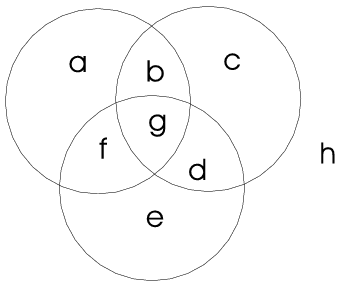Mathematical and Physical Journal
for High Schools
Issued by the MATFUND Foundation
 Already signed up? New to KöMaL?

#Problem C. 860. (September 2006)

C. 860. A survey carried out on a sample of 500 revealed that 46% of those asked liked strawberry ice cream, 71% liked vanilla and 85% liked chocolate ice cream. Is there anyone in the sample who likes all three flavours?

(5 pont)

Deadline expired on October 16, 2006.

Sorry, the solution is available only in Hungarian. Google translation

Megoldás: Van.A Venn-diagram alapján a következő egyenleteket írhatjuk föl:

a+b+c+d+e+f+g+h=500,

a+b+f+g=0,46.500=230,

b+c+d+g=0,71.500=355,

d+e+f+g=0,85.500=425.

Ezek alapján

500-(230+355+425)=-510=(a+b+c+d+e+f+g+h)-(a+b+f+g)-(b+c+d+g)-(d+e+f+g)=h-(b+d+f+2g).

Vagyis (b+d+f+g)+g=500+(10+h), és mivel b+d+f+g legfeljebb 500, a jobboldal viszont legalább 510, ezért g legalább 10. Vagyis legalább 10 olyan ember van, aki mind a háromféle fagylaltot szereti.

### Statistics:

 683 students sent a solution. 5 points: 577 students. 4 points: 13 students. 3 points: 25 students. 2 points: 15 students. 1 point: 9 students. 0 point: 34 students. Unfair, not evaluated: 10 solutionss.

Problems in Mathematics of KöMaL, September 2006